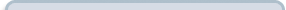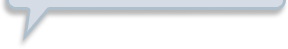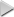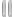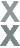# Prize Winner, Part 3

###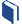Resources for this lesson: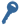Key Term

Theoretical probability

You will use your Algebra II Journalon this page.

> Glossary> Calculator Resources> Teacher Resources: Instructional NotesAs you can see, having the letters in the game repeat makes for a much more challenging game. Let’s keep the game the way it was originally designed, with three doors and the possibility of the letters repeating.

What’s Behind the Door?

If you can guess the letter behind the door, you win a prize!

Large Prize:
Guess all three doors correctly

Medium Prize:
Guess two doors correctly

Small Prize:
Guess one door correctly

Prize

Probability to Win

Small

$\frac{12}{27}$

Medium

$\frac{6}{27}$

Large

$\frac{1}{27}$

These probabilities are the theoretical probabilities. Theoretically, a person should win a small prize twice as often as a medium prize, and approximately 50% of the time a contestant wins a small prize. Seldom should a contestant win a large prize.

Let’s conduct a probability simulation to see if these theoretical probabilities hold true!

##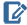Algebra II Journal: Reflection 1

Use the following simulation of the Guess the Letter Behind the Door game. Run the simulation for at least 50 trials. Record the results in your Algebra II Journaland submit to your teacher.